# Hidden patients and hidden genes: Understanding cancer data with matrix factorization¶

Biotechnological innovations in the last decade have enabled systematic large-scale cancer genome studies. Cancer projects such as The Cancer Genome Atlas (TCGA) and The International Cancer Genome Consortium (ICGC) coordinate the generation of catalogues of tumors from over 50 different cancer types that are of clinical importance across the globe. These catalogues contain comprehensive data of more than 25,000 cancer genomes at a high, often at a single nucleotide, resolution level. The genomes of individual cancers are accompanied by the analyses of transcriptome, epigenome and sequence variation. Furthermore, where possible, measurements are provided for matched tumor and non-tumor tissue to distinguish inherited from oncogenic variation.

Diversity and abundance of data provided by the cancer projects challenge computer scientists of all kinds to develop innovative software, hardware and analytic solutions for data analysis. The hope is that with the computationally and statistically stronger approaches we will be able to reveal biological features that drive cancer development, define cancer types relevant for prognosis and, ultimately, enable the development of new cancer therapies.

Here, we analyze a transcriptome data set on breast cancer obtained from the ICGC. The analysis relies on a popular class of data mining techniques, called nonnegative matrix factorization, to find groups of patients with similar expression profiles and groups of genes with similar activation levels across patients.

In :
%matplotlib inline

from collections import defaultdict, Counter
import urllib

import numpy as np
from matplotlib import pyplot as plt
import matplotlib.gridspec as gridspec
from sklearn import preprocessing
import scipy.cluster.hierarchy as sch

import nimfa


## Obtaining data from the International Cancer Genome Consortium (ICGC)¶

We start by downloading data from the ICGC Data Portal (https://dcc.icgc.org/projects). In addition to its data repository role, the ICGC Data Portal also provides tools for visualizing and querying the data, for example, a distribution of somatic mutations in patient's genomes across cancer types. In this column we use publicly available data, however, we note that researchers have to apply for access to controlled data.

In :
clinical_fname = 'clinical.BRCA-US.tsv.gz'
'fn=/release_18/Projects/BRCA-US/' \
'clinical.BRCA-US.tsv.gz', clinical_fname);

expression_fname = 'protein_expression.BRCA-US.tsv.gz'
'fn=/release_18/Projects/BRCA-US/' \
'protein_expression.BRCA-US.tsv.gz', expression_fname);


Once the download has completed, we have two new files on our local disk. The first file, clinical.BRCA-US.tsv.gz, contains basic clinical information, such as patient and specimen identifiers and categorization of specimens into tumor and non-tumor types. The second file, protein_expression.BRCA-US.tsv.gz, is a protein expression data set of breast cancer patients (BRCA is a standard acronym used by the cancer projects to indicate data related to breast cancer). Each row in the expression file contains normalized expression value of a particular protein in a given specimen of a specific patient.

we read data columns that correspond to patient and specimen identifiers and expression measurements into two numpy arrays, C and E.

In :
C = np.genfromtxt(clinical_fname, delimiter='\t', dtype='object',

In :
E = np.genfromtxt(expression_fname, delimiter='\t', dtype='object',

In :
donors = set(E[:, 0])
genes = set(E[:, 2])
print(len(donors))
print(len(genes))

298
115


## Data preprocessing¶

In :
spec2gene = defaultdict(set)
for spec_id, gene in E[:, 1:3]:

donor2spec = defaultdict(set)
for donor, spec_id in E[:, :2]:

# how many specimens are there per donor?
tmp = [len(v) for v in donor2spec.values()]
print(Counter(tmp))

# protein expression data is available only for tumor data (no matched normal tissue)
spec2spec_type = {C[idx, 1]: C[idx, 2] for idx, spec in enumerate(C[:, 1]) if spec in spec2gene}
print(set(spec2spec_type.values()))

Counter({1: 298})
{b'Primary tumour - solid tissue'}

In :
data = np.zeros((len(donors), len(genes)))

donor2id = {donor.decode('UTF-8'): idx for idx, donor in enumerate(donors)}
id2donor = dict(zip(donor2id.values(), donor2id.keys()))

gene2id = {gene.decode('UTF-8'): idx for idx, gene in enumerate(genes)}
id2gene = dict(zip(gene2id.values(), gene2id.keys()))

for donor, gene, val in E[:, [0, 2, 3]]:
data[donor2id[donor.decode('UTF-8')], gene2id[gene.decode('UTF-8')]] = float(val)

In :
# level of sparsity
print(np.sum(data != 0)/data.size)

# negative elements
print(np.sum(data < 0))

# remove negative elements
data = data - np.min(data)

# normalize data
data = preprocessing.Normalizer().fit_transform(data)

1.0
17147


## Clustering of genes¶

Next, we perform cluster analysis to identify groups of genes with similar behavior across patients. The hypothesis is that genomes of all cancers accumulate somatic and epigenetic marks that affect protein-coding or regulatory components of genes. These marks in turn silence or overexpress genes, which results in notable changes of protein expression levels in tumor tissue. By finding genes that are consistently silenced or overexpressed in cancer, we might narrow down candidate genes and provide focus for downstream analysis.

### Nonnegative matrix factorization¶

Nonnegative matrix factorization (NMF) is typically concerned with the decomposition of a nonnegative data matrix into two low-dimensional (latent) data matrices, such that their matrix product provides a good surrogate of the original data matrix.

There are a few important concepts to understand in order to fully appreciate the NMF. First, since not only input data matrix is nonnegative, but also the latent matrices contain solely nonnegative values, NMF is able to learn a parts-based data representation. For example, if we apply NMF to a database of facial images, then the latent components (vectors) of the matrices estimated by the NMF correspond to parts of faces. If we applied NMF to a text corpus, then the latent components would encode semantic features of text. Second, high generalization power of NMF is due to its ability to compress large input data matrix and represent it with latent matrices, which have substantially fewer dimensions. Technically, the dimensionality of latent matrices is known as factorization rank. In this sense, NMF might be a good example where less can be more.

Many variants of nonnegative matrix factorization have been developed in recent years. These augment the objective function with different types of regularization imposed on the latent matrices, provide various generalizations of the notion of a good matrix surrogate, and extend factorization of a single data matrix to simultaneous decomposition of multiple matrices.

We consider Nimfa, an open-source Python library for NMF, to factorize our matrix of protein expression measurements. Nimfa implements over a dozen of nonnegative matrix factorization algorithms, initialization techniques and provides quality scoring.

Zitnik, Marinka, and Blaz Zupan. Nimfa: A Python library for nonnegative matrix factorization. The Journal of Machine Learning Research 13, 1:849-853, 2012.



In our example, we run one of the most widely used NMF variants. This variant estimates the NMF model by iteratively improving current estimates of the latent matrices until a local minimum is attained, measured as Kullback-Leibler divergence between the input matrix and its estimated matrix surrogate.

In :
rank = 10
nmf = nimfa.Nmf(data, rank=rank, seed='random_vcol', max_iter=100,
update='divergence', objective='div', n_run=50, track_factor=True)
nmf_fit = nmf()


### Plot dendrogram along with the heatmap¶

Next, we use the estimated NMF model to cluster genes into groups. Whereas the latent components obtained from an image database correspond to parts of faces, the latent components of a protein expression data set represent subpopulations of patients and gene subsets. Conveniently, we refer to these latent components as hidden patients and hidden genes.

We now use the memberships of genes to hidden genes as a means to group genes together. Notice that above we ran the NMF algorithm 50 times (n_run = 50). To obtain a stable gene clustering we examine gene-hidden gene memberships from all factorization runs and average gene membership indicators to obtain a consensus clustering.

Once we obtain the consensus clustering we can visualize it in a heatmap with the rows reordered to make the groups explicit.

In :
def clean_axis(ax):
ax.get_xaxis().set_ticks([])
ax.get_yaxis().set_ticks([])
for sp in ax.spines.values():
sp.set_visible(False)

fig = plt.figure(figsize=(13.9, 10))
heatmapGS = gridspec.GridSpec(1, 2, wspace=.01, hspace=0., width_ratios=[0.25,1])

C = 1 - nmf_fit.fit.consensus()

denD = sch.dendrogram(Y, orientation='right', link_color_func=lambda k: 'black')
clean_axis(denAX)

D = C[denD['leaves'], :][:, denD['leaves']]
axi = heatmapAX.imshow(D, interpolation='nearest', aspect='equal', origin='lower', cmap='RdBu')
clean_axis(heatmapAX)

cb = fig.colorbar(axi, fraction=0.046, pad=0.04, aspect=10)
cb.set_label('Distance', fontsize=20)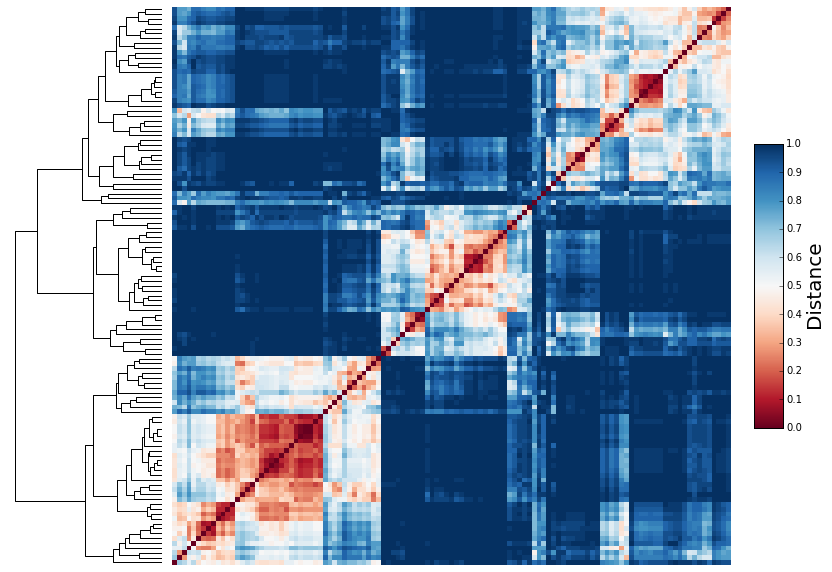## Clustering of patients¶

Similarly, we can stratify cancer patients into groups by considering memberships of patients to the respective latent components (i.e., hidden patients).

### Nonnegative matrix factorization¶

In :
rank = 10
nmf = nimfa.Nmf(data.T, rank=rank, seed='random_vcol', max_iter=200,
update='euclidean', objective='conn', conn_change=40,
n_run=50, track_factor=True)
nmf_fit = nmf()


### Plot dendrogram along with the heatmap¶

In :
def clean_axis(ax):
ax.get_xaxis().set_ticks([])
ax.get_yaxis().set_ticks([])
for sp in ax.spines.values():
sp.set_visible(False)

fig = plt.figure(figsize=(13.9, 10))
heatmapGS = gridspec.GridSpec(1, 2, wspace=.01, hspace=0., width_ratios=[0.25,1])

C = 1 - nmf_fit.fit.consensus()

denD = sch.dendrogram(Y, orientation='right', link_color_func=lambda k: 'black')
clean_axis(denAX)

D = C[denD['leaves'], :][:, denD['leaves']]
axi = heatmapAX.imshow(D, interpolation='nearest', aspect='equal', origin='lower', cmap='RdBu')
clean_axis(heatmapAX)

cb = fig.colorbar(axi, fraction=0.046, pad=0.04, aspect=10)
cb.set_label('Distance', fontsize=20)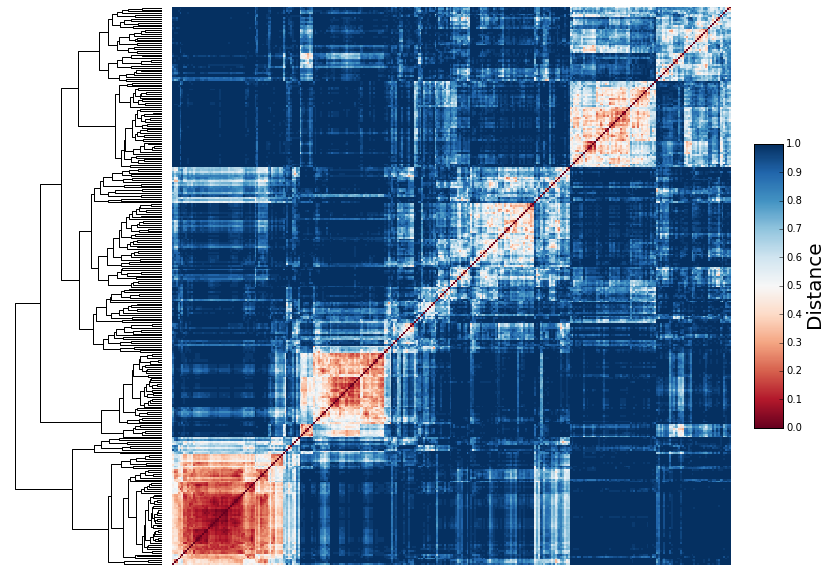## Hidden patients and hidden genes¶

### Latent dimensionality selection¶

In :
rank_cands = range(3, 30, 5)
snmf = nimfa.Snmf(data, seed='random_vcol', max_iter=100)
summary = snmf.estimate_rank(rank_range=rank_cands, n_run=10, what='all')

In :
summary.keys()

Out:
dict_keys(['euclidean', 'dispersion', 'cophenetic', 'rss', 'residuals', 'kl', 'connectivity', 'select_features', 'sparseness', 'n_run', 'n_iter', 'rank', 'consensus', 'evar', 'score_features', 'predict_samples', 'predict_features'])
In :
rss = [summary[rank]['rss'] for rank in rank_cands]
coph = [summary[rank]['cophenetic'] for rank in rank_cands]
disp = [summary[rank]['dispersion'] for rank in rank_cands]
spar = [summary[rank]['sparseness'] for rank in rank_cands]
spar_w, spar_h = zip(*spar)
evar = [summary[rank]['evar'] for rank in rank_cands]

plt.plot(rank_cands, coph, 'o-', label='Cophenetic correlation', linewidth=2)
plt.plot(rank_cands, disp,'o-', label='Dispersion', linewidth=2)
plt.plot(rank_cands, spar_w, 'o-', label='Sparsity (Basis)', linewidth=2)
plt.plot(rank_cands, spar_h, 'o-', label='Sparsity (Mixture)', linewidth=2)
plt.plot(rank_cands, evar, 'o-', label='Explained variance', linewidth=2)
plt.legend(loc='upper center', bbox_to_anchor=(0.5, -0.05), ncol=3, numpoints=1);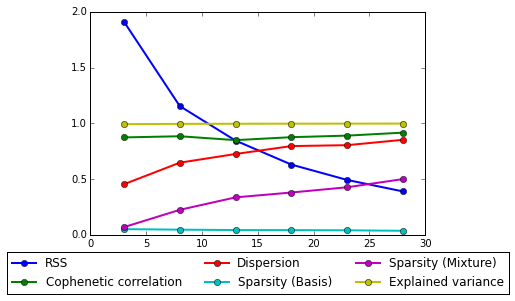### A hidden gene¶

Recall that a hidden gene corresponds to a latent component (a vector) in the low-dimensional matrices estimated by the NMF. We would like to know, which genes are most strongly associated with a particular hidden gene.

#### Nonnegative matrix factorization¶

In :
# SNMF/L: for sparse W (where ‘l’ denotes the sparseness imposed on the left factor)
# SNMF/R: for sparse H (where ‘r’ denotes the sparseness imposed on the right factor)
rank = 13
snmf = nimfa.Snmf(data.T, rank=rank, seed='random_vcol', version='l', max_iter=200)
snmf_fit = snmf()


#### Genes associated with a hidden gene¶

In :
W = snmf_fit.fit.basis()

c = 0
k = 10

topk = np.argsort(np.asarray(W[:, c]).flatten())[-k:]
val = W[topk, c]

plt.barh(np.arange(k) + .5, val, align="center")
labels = [id2gene[idx] for idx in topk]
plt.yticks(np.arange(k) + .5, labels)
plt.xlabel("Weight")
plt.ylabel("Gene");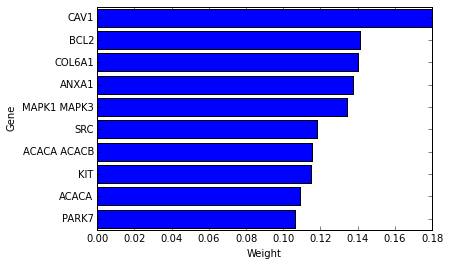#### The functional content of a hidden gene¶

In :
print('\n'.join(labels))

PARK7
ACACA
KIT
ACACA ACACB
SRC
MAPK1 MAPK3
ANXA1
COL6A1
BCL2
CAV1


Go to the website http://geneontology.org and perform the enrichment analysis. This tells you whether members of the gene set share functional roles in the cell, participate together in biological processes, and if the strength of gene-gene association is stronger than what would be expected if genes were picked randomly.

#### Gene scoring and selection¶

A detailed explanation of gene scoring and selection is provided in

Kim, Hyunsoo, and Haesun Park. Sparse non-negative matrix factorizations via alternating non-negativity-constrained least squares for microarray data analysis. Bioinformatics 23, 12:1495-1502, 2007.
In :
gs = snmf_fit.fit.score_features()

In :
gss = snmf_fit.fit.select_features()
gene_set = [id2gene[idx] for idx in np.nonzero(gss)]

In :
print('\n'.join(gene_set))

ANXA1
GATA3
FN1
MAPK1 MAPK3
PRKAA1
GSK3A GSK3B
ACACA ACACB
CDH1
PDCD4
STAT5A
HSPA1A
CAV1
COL6A1
AR
IGFBP2
SCD1
CTNNB1
CASP7
PGR
ACACA
BCL2
CLDN7
CCNB1
SERPINE1
KIT


Go to the website http://geneontology.org and perform the enrichment analysis. This tells you whether members of the gene set share functional roles in the cell, participate together in biological processes, and if the strength of gene-gene association is stronger than what would be expected if genes were picked randomly.

### A hidden patient¶

Recall that a hidden patient is a latent component (a vector) of the low-dimensional matrices estimated by the NMF. We would like to know, which patient are most strongly associated with a particular hidden patient.

#### Nonnegative matrix factorization¶

In :
# SNMF/L: for sparse W (where ‘l’ denotes the sparseness imposed on the left factor)
# SNMF/R: for sparse H (where ‘r’ denotes the sparseness imposed on the right factor)
rank = 13
snmf = nimfa.Snmf(data.T, rank=rank, seed='random_vcol', version='r', max_iter=200)
snmf_fit = snmf()


#### Patients associated with a hidden patient¶

In :
H = snmf_fit.fit.coef().T

c = 0
k = 30

topk = np.argsort(np.asarray(H[:, c]).flatten())[-k:]
val = H[topk, c]

plt.barh(np.arange(k) + .5, val, color="yellow", align="center")
labels = [id2donor[idx] for idx in topk]
plt.yticks(np.arange(k) + .5, labels)
plt.xlabel("Weight")
plt.ylabel("Donor");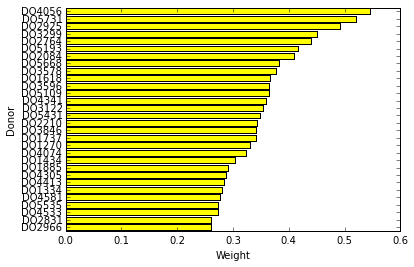In [ ]: